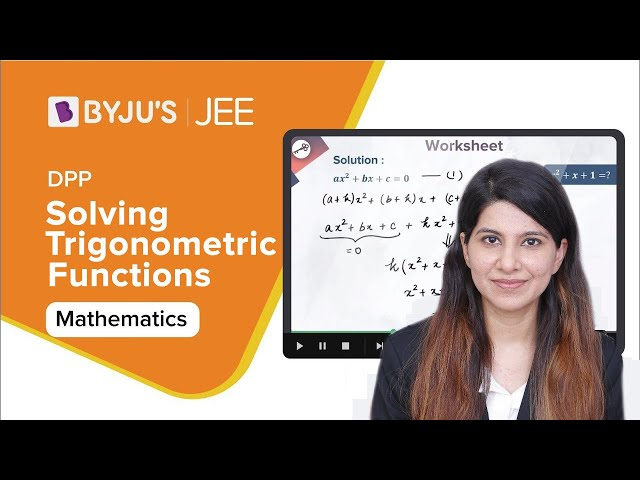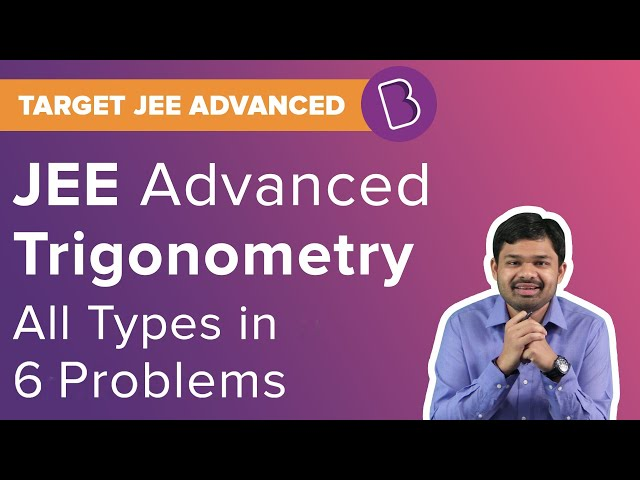# JEE Main Maths Trigonometry Previous Year Questions With Solutions

Trigonometry is important to mathematics as it involves the study of calculus, statistics and linear algebra. This article covers the identities of trigonometry and trigonometric equations, functions of trigonometry, properties of inverse trigonometric functions, problems on heights and distances.

The trigonometry questions from the previous years of JEE Main are present on this page, along with the detailed solution for each question. About 2-3 questions are asked from this topic in JEE Examination.

## What is Trigonometry?

Trigonometry is an essential element of calculus. In statistics, trigonometric functions are needed for the calculation of the bell curve and for the study of periodic periodicities. The other uses of trigonometry in different fields relate to the use of the Fourier series, which cannot be computed without the use of trigonometry.

Trigonometry in general deals with the study of the relationships concerning angles and the lengths of triangles. The two branches of trigonometry are:

• Plane Trigonometry
• Spherical Trigonometry

Plane trigonometry concentrates on the connection between the sides of triangles and angles. Students learn about theories like right angles, acute angles, straight angles, complementary angles and supplementary angles. Considering the triangles are all situated on a plane, the sum of the angles is always 180 degrees.

In spherical trigonometry, students learn about curved triangles drawn on the surface of a sphere. This refers to the sum of the angles of these triangles is higher than 180 degrees. Spherical trigonometry is used in astronomy and navigation.

## Trigonometry Videos

### Solving-Trigonometric-Functions### JEE Trigonometry## JEE Main Maths Trigonometry Previous Year Questions With Solutions

Question 1: The general solution of sin x − 3 sin2x + sin3x = cos x − 3 cos2x + cos3x is _________.

Solution:

sinx − 3 sin2x + sin3x = cosx − 3 cos2x + cos3x

⇒ 2 sin2x cosx − 3 sin2x − 2 cos2x cosx + 3 cos2x = 0

⇒ sin2x (2cosx − 3) − cos2x (2 cosx − 3) = 0

⇒ (sin2x − cos2x) (2 cosx − 3) = 0

⇒ sin2x = cos2x

⇒ 2x = 2nπ ± (π / 2 − 2x) i.e.,

x = nπ / 2 + π / 8

Question 2: If sec 4θ − sec 2θ = 2, then the general value of θ is __________.

Solution:

sec 4θ − sec 2θ = 2 ⇒ cos 2θ − cos 4θ = 2 cos 4θ cos 2θ

⇒ −cos 4θ = cos 6θ

⇒ 2 cos 5θ cosθ = 0

⇒ H = [h cot 15o] / [cot 15o − 1] or

nπ/5 + π/10

Question 3: If tan (cot x) = cot (tan x), then sin 2x = ___________.

Solution:

tan (cotx) = cot (tanx) ⇒ tan (cotx) = tan (π / 2 − tanx)

cotx = nπ + π / 2 − tanx

⇒ cotx + tanx = nπ + π / 2

2 sin2x = nπ + π / 2

⇒ sin2x = 2 / [nπ + {π / 2}]

= 4 / {(2n + 1) π}

Question 4: If the solution for θ of cospθ + cosqθ = 0, p > 0, q > 0 are in A.P., then numerically the smallest common difference of A.P. is ___________.

Solution:

Given cospθ = −cosqθ = cos (π + qθ)

pθ = 2nπ ± (π + qθ), n ∈ I

θ = [(2n + 1)π] / [p − q] or [(2n − 1)π] / [p + q], n ∈ I

Both the solutions form an A.P. θ = [(2n + 1)π] / [p − q] gives us an A.P. with common difference 2π / [p − q] and θ = [(2n − 1)π] / [p + q] gives us an A.P. with common difference = 2π / [p + q].

Certainly, {2π / [p + q]} < {∣2π / [p − q]∣}.

Question 5: If a, b are different values of x satisfying a cosx + b sinx = c, then tan ([α + β] / 2) = ______________.

Solution:

a cosx + b sinx = c ⇒ a {[(1 − tan2 (x / 2)] / [1 + tan2 (x / 2)]} + 2b {[tan (x / 2) / 1 + tan2 (x / 2)} = c

⇒ (a + c) * tan2 [x / 2] − 2b tan [x / 2] + (c − a) = 0

This equation has roots tan [α / 2] and tan [β / 2].

Therefore, tan [α / 2] + tan [β / 2] = 2b / [a + c] and tan [α / 2] * tan [β / 2] = [c − a] / [a + c] Now

tan (α / 2 + β / 2) = {tan [α / 2] + tan [β / 2]} / {1 − tan [α / 2] * tan [β / 2]} = {[2b] / [a + c]} / {1− ([c − a] / [a + c])} = ba

Question 6: In a triangle, the length of the two larger sides are 10 cm and 9 cm, respectively. If the angles of the triangle are in arithmetic progression, then the length of the third side in cm can be _________.

Solution:

We know that in a triangle larger the side, larger the angle.

Since angles ∠A, ∠B and ∠C are in AP.

Hence, ∠B = 60o cosB = [a2 + c2 −b2] / [2ac]

⇒ 1 / 2 = [100 + a2 − 81] / [20a]

⇒ a2 + 19 = 10a

⇒ a2 − 10a + 19 = 0

a = 10 ± (√[100 − 76] / )

⇒ a + c√2 = 5 ± √6

Question 7: In triangle ABC, if ∠A = 45∘, ∠B = 75∘, then a + c√2 = __________.

Solution:

∠C = 180o − 45o − 75o = 60o

Therefore, a + c√2 = k (sin A + √2 sinC)

= k (1 / √2 + [√3 / 2] * √2)

= k ([1 + √3] / [√2]) and

k = b / [sin B]

⇒ a + c√2= b / sin75o ([1 + √3] / [√2])

= 2b

Question 8: If cos−1 p + cos−1 q + cos−1 r = π then p2 + q2 + r2 + 2pqr = ________.

Solution:

According to given condition, we put p = q = r = 1 / 2

Then, p2 + q2 + r2 + 2pqr = (1 / 2)2 + (1 / 2)2 + (1 / 2)2 + 2 * [1 / 2] * [1 / 2] * [1 / 2]

= [1 / 4] + [1 / 4] + [1 / 4] + [2 / 8]

=1

Question 9: tan [(π / 4) + (1 / 2) * cos−1 (a / b)] + tan [(π / 4) − (1 / 2) cos−1 .(a / b)] = _________.

Solution:

tan [(π / 4) + (1 / 2) * cos−1 (a / b)] + tan [(π / 4) − (1 / 2) cos−1 .(a / b)]

Let (1 / 2) * cos−1 (a / b) = θ

⇒ cos 2θ = a / b

Thus, tan [{π / 4} + θ] + tan [{π / 4} − θ] = [(1 + tanθ) / (1 − tanθ)]+ ([1 − tanθ] / [1 + tanθ])

= [(1 + tanθ)2 + (1 − tanθ)2] / [(1 − tan2θ)]

= [1 + tan2θ + 2tanθ + 1 + tan2θ − 2tanθ] / [(1 + tan2θ)]

= 2 (1 + tan2θ) / [(1 + tan2θ)]

= 2 sec2θ

= 2 cos2θ

= 2 / [a / b]

= 2b / a

Question 10: The number of real solutions of tan−1 √[x (x + 1)] + sin−1 [√x2 + x + 1] = π / 2 is ___________.

Solution:

tan−1 √[x (x + 1)] + sin−1 [√x2 + x + 1] = π / 2

tan−1 √[x (x + 1)] is defined when x (x + 1) ≥ 0 ..(i)

sin−1 [√x2 + x + 1] is defined when 0 ≤ x (x + 1) + 1 ≤ 1 or 0 ≤ x (x + 1) ≤ 0 ..(ii)

From (i) and (ii), x (x + 1) = 0 or x = 0 and -1.

Hence, the number of solution is 2.

Question 11: What is the value of sin (cot−1 x)?

Solution:

Let cot−1 x = θ ⇒ x = cotθ

Now cosecθ = √[1 + cot2θ] = √[1 + x2]

Therefore, sinθ = 1 / cosecθ

= 1 / √[1 + x2]

⇒θ = sin−1 {1 / √[1 + x2]}

Hence, sin (cot−1 x) = sin (sin−1 {1 / √[1 + x2]})

= 1 / √[1 + x2]

= (1 + x2)−1/2

Question 12: sec2 (tan−1 2) + cosec2 (cot−1 3) = _________.

Solution:

Let (tan−1 2) = α

⇒ tan α = 2 and cot−1 3 = β

⇒ cot β = 3 sec2 (tan−1 2) + cosec2 (cot−1 3)

= sec2 α + cosec2 α

= 1 + tan2α + 1 + cot2α

= 2 + (2)2 + (3)2

= 15

Question 13: A vertical pole consists of two parts, the lower part being one-third of the whole. At a point in the horizontal plane through the base of the pole and distance 20 meters from it, the upper part of the pole subtends an angle whose tangent is 1 / 2. The possible heights of the pole are _________.

Solution:

[H / 3] cotα = d and d = 150 cotφ = 60m or

[H / 3d] = tanα and

[H / d] = tanβ

tan (β − α) = 1 / 2

= {[H / d] − [H / 3d]} / {1 + [H2 / 3d2]}

⇒ 1 + [H2 / 3d2] = 4H / 3d

⇒ H2 − 4dH + 3d2 = 0

⇒ H2 − 80H + 3 * (400) = 0

⇒ H = 20 or 60m

Question 14: A tower of height b subtends an angle at a point O on the level of the foot of the tower and a distance a from the foot of the tower. If a pole mounted on the tower also subtends an equal angle at O, the height of the pole is ___________.

Solution:

tanα = b / a, tan2α = [2 (b / a)] / [1 − (b / a)2] = [p + b] / a

⇒ 2ba / [a2 − b2] = [p + b] / a

⇒ [2ba2 − a2b + b3] / [a2 − b2] = p

⇒ p = [b * (a2 + b2)] / [a2 − b2]

Question 15: A balloon is observed simultaneously from three points A, B and C on a straight road directly under it. The angular elevation at B is twice and at C is thrice that of A. If the distance between A and B is 200 metres and the distance between B and C is 100 metres, then the height of balloon is given by _________.

Solution:

x = h cot3α …..(i)

(x + 100) = h cot2α ……(ii)

(x + 300) = h cotα ……(iii)

From (i) and (ii), −100 = h (cot3α − cot2α),

From (ii) and (iii), −200 = h (cot2α − cotα),

100 = h ([sinα] / [sin3α * sin2α]) and 200 = h ([sinα] / [sin2α * sinα]) or

sin3α / sinα = 200 / 100

⇒ sin3α / sinα = 2

⇒ 3 sinα − 4 sin3α − 2 sinα = 0

⇒ 4 sin3α − sinα = 0

⇒ sinα = 0 or

sin2α = 1 / 4 = sin2 (π / 6)

⇒ α = π / 6

Hence, h = 200 * sin [π / 3]

= 200* [√3 / 2]

Test Your Knowledge On Maths Trigonometry Previous Year Questions With Solutions!